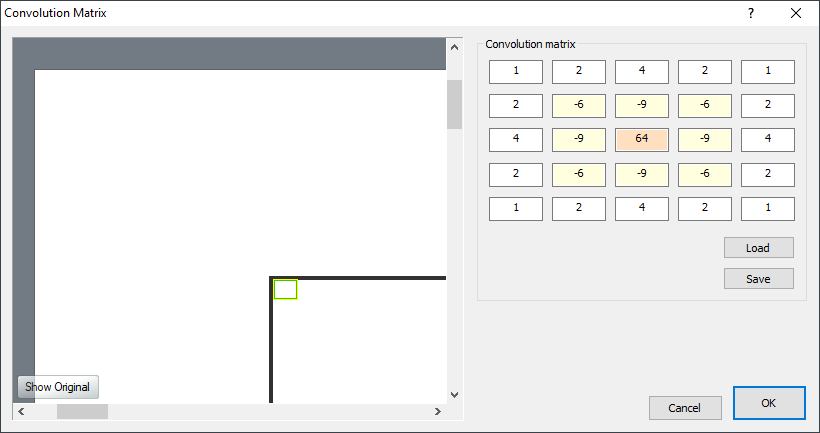...

Chasys Draw IES Help:Effects : Custom via Convolution Matrix Introduction The Convolution Matrix loops through all pixels, adding up the current pixel with the surrounding pixels using the values provided in the matrix as weights for the addition. Put differently; for each pixel, it uses the center value in the matrix as the value of the current pixel being manipulated. For example, in a 3 x 3 matrix, the center value is at (1, 1). It then multiplies the values from the matrix by the surrounding pixels and adds the resulting values for all pixels to get the value for the resulting center pixel. The input matrix is a 5-by-5 matrix, centered at (2, 2):You will be provided with a dialog box in which you specify the elements of the convolution matrix to be used to implement the effect. In case you are wondering why there isn't a "scale" parameter like in other image editors, Chasys Draw IES Artist knows how to calculate that. Storage and Retrieval The matrices can be stored to and retrieved from files; for this, two formats are supported, JpCHA2 Matrix format (.MAT) files containing a 5x5 matrices and Adobe Custom Filter (.ACF) files. The default matrix is the "SINC SPREAD". Used on human faces, this enhances hair while suppressing wider artifacts like pimples, giving the impression that the image is of a higher resolution than it actually is. Try it!